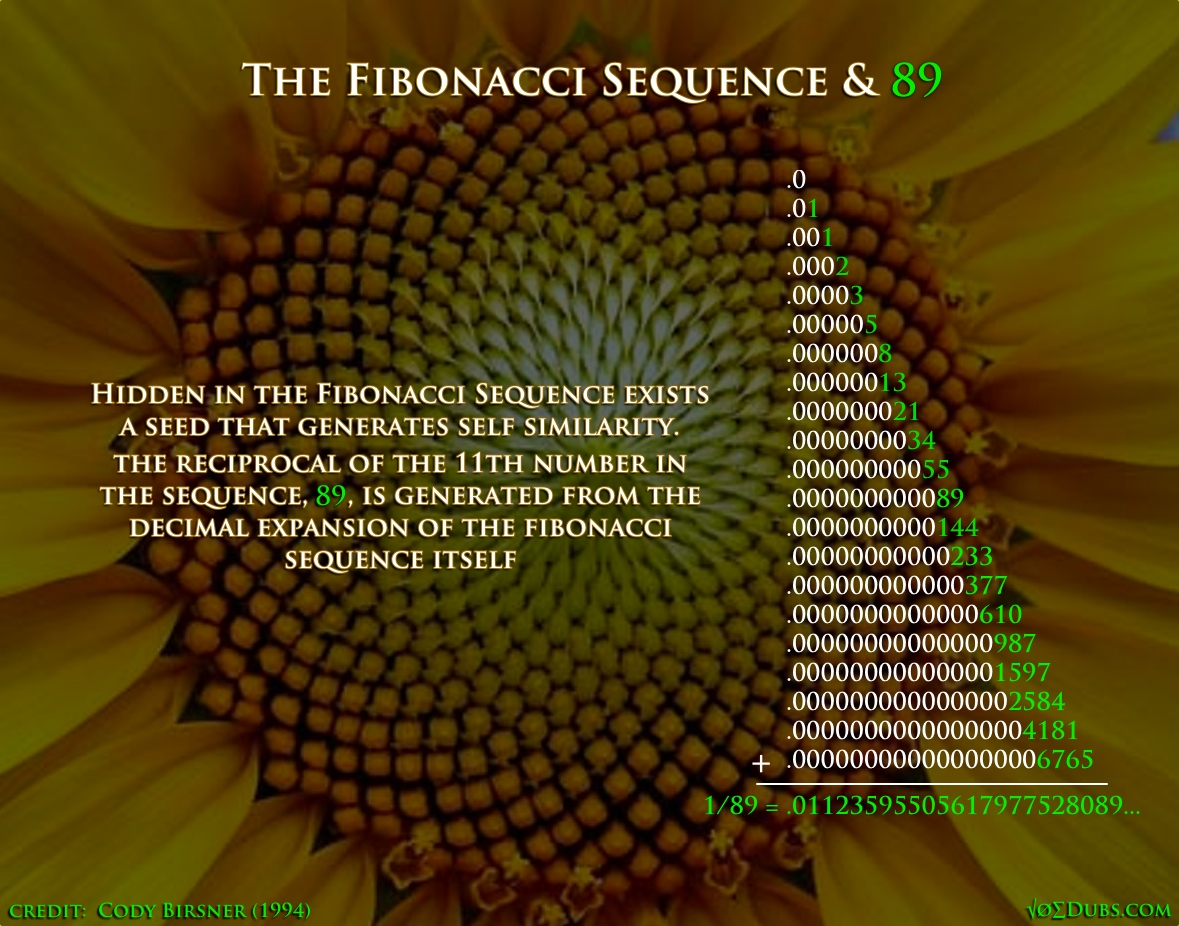## Reciprocal GnosisTherein lies esoteric gnosis of the inner-workings of reality through analyzing the first principle of the Quadrivium, Number.  The reciprocals of the natural numbers reveal patterns that are visible only when we put on our goggles of decimal expansion and peer deep into the realms of numeric possibilities. The reciprocal of a number is simply one divided by that number. The reciprocal of 5 is .2 because 1/5 = .2  Conversely, the reciprocal of 2 is 5, because 1/5 = .2 This resembles patterns that arise is what is known as vortex based math.  The reciprocals of certain numbers reveal patterns that are easily recognizable at first, but then disintegrate into the apparent randomness. But it’s not random. There exists intelligence in the way nature operates.  With this reciprocal gnosis we can analyze the quality of Number itself and reveal order from ostensible chaos. The reciprocal of 49 (1/49) reveals binary numbers,…

10

## Cosmic ProportionsThe physical sizes of Earth and Moon are encoded into the proportions and measurements we commonly use in our daily lives.  From Renaissance artists to 21st century school teachers, we all use these cosmic proportions.  Very few are cognizant of their underlying meaning. If our Moon sat directly tangent to Earth, these are the proportions that arise.  The circumference of the circle is equal to the perimeter of the square to 99.97% accuracy according to NASA’s own data. The Great Pyramid’s dimensions can be broken down to 7 high, and 11 across.  The perimeter of the base equals the circumference of a circle whose radius is equal to the height of the pyramid. The Moon and Earth can be summed up amazingly well using the numbers 3 and 11.  Maybe that punk rock band understood these sacred geometrical concepts and hid it in their name.

5

## Fibonacci: The Fractions of LifeThe Fibonacci Sequence is Nature’s favorite series of numbers.  They are found wherever there is life.  Closely associated with phi, this sequence actually generates this golden ratio when any number is divided by the number before it. Phi = 1.618033988.. The Fibonacci Sequence: 0, 1, 1, 2, 3, 5, 8, 13, 21, 34, 55, 89, 144, 233, 377, 610, 1597, 2584, 4181, 6765, 10946, 17711, 28657, 46368, … Phi and phee are generated from the Fibonacci sequence in this way.  This might be an indication that fractions are important, especially in relation to the Fibonacci numbers, since they create the golden ratio or the divine proportion. The 11th number in the sequence is 89.  Zero is not a number but a placeholder for numeric expansion and contraction.  Interestingly 89 is 11 less than one hundred, but even more interesting is its reciprocal, which is 1/89.…

3